Processing ......FreeComputerBooks.com Links to Free Computer, Mathematics, Technical Books all over the World

An Introduction to Gödel’s Theorems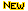Top Free Algorithms Books - 100% Free or Open Source!
• Title: An Introduction to Gödel’s Theorems
• Author(s) Peter Smith
• Publisher: Independently published (August 19, 2020)
• Paperback: 402 pages
• eBook: PDF (402 pages)
• Language: English
• ISBN-10/ASIN: B08GB4L9JT
• ISBN-13: 979-8673862131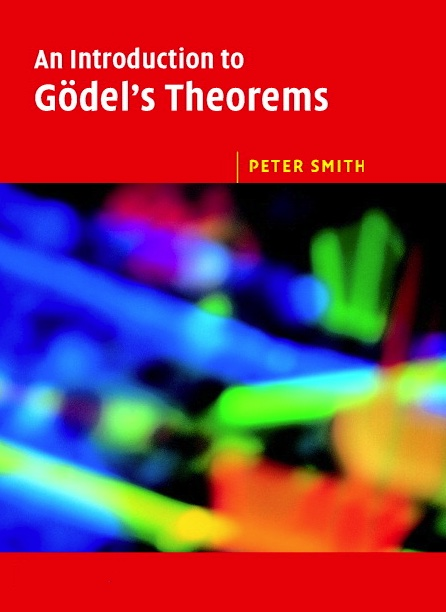Book Description

The formal explanations are interwoven with discussions of the wider significance of the two Theorems. This book -- extensively rewritten for its second edition -- will be accessible to philosophy students with a limited formal background. It will be of equal interest to mathematics students taking a first course in mathematical logic.

• Until he retired, Peter Smith taught logic at the University of Cambridge. His Logic Matters website and blog is at https://www.logicmatters.net.
Reviews, Ratings, and Recommendations: Related Book Categories: Read and Download Links:Similar Books:
•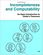Incompleteness and Computability: Gödel's Theorems

This book is an introduction to metamathematics and Gödel's Theorems. It covers recursive function theory, arithmetization of syntax, the first and second incompleteness theorem, models of arithmetic, second-order logic, and the lambda calculus.

•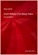Gödel Without (Too Many) Tears (Peter Smith)

How is this remarkable result of Gödel's Theorems established? This short book explains. The aim is to make the Theorems available, clearly and accessibly, even to those with a quite limited formal background.

•What is Mathematics: Godel's Theorem and Around (K. Podnieks)

This accessible book gives a new, detailed and elementary explanation of the Godel incompleteness theorems and presents the Chaitin results and their relation to the da Costa-Doria results, which are given in full, but with no technicalities.

•Mathematics in the Age of the Turing Machine (Thomas C. Hales)

Computers have rapidly become so pervasive in mathematics that future generations may look back to this day as a golden dawn. The article gives a survey of mathematical proofs that rely on computer calculations and formal proofs.

•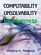Computability, Unsolvability, Randomness (Stephen G. Simpson)

The author exposits Turing's 1936 theory of computability and unsolvability, as subsequently developed by Kleene and Post. This theory is of the essence in theoretical computer science and in the study of unsolvable mathematical problems.

•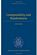Computability and Randomness (Andre Nies)

The complexity and the randomness aspect of a set of natural numbers are closely related. This book includes a detailed treatment of Turing's theory of computability and unsolvability as subsequently developed by Kleene, Post, Friedberg, etc.

•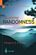Exploring Randomness (Gregory J. Chaitin)

This book presents the technical core of the theory of program-size complexity. LISP is used to present the key algorithms and to enable computer users to interact with the authors proofs and discover for themselves how they work.

•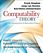Computability Theory: Introduction to Recursion Theory

Computability Theory originated with the seminal work of Gödel, Church, Turing, Kleene and Post in the 1930s. These texts provide concise, comprehensive, and authoritative introduction to contemporary computability theory, techniques, and results.

•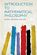Introduction to Mathematical Philosophy (Bertrand Russell)

Requiring neither prior knowledge of mathematics nor aptitude for mathematical symbolism, the book serves as essential reading for anyone interested in the intersection of mathematics and logic and in the development of analytic philosophy.

•Introduction to Mathematical Logic (Vilnis Detlovs, et al)

This book explores the principal topics of mathematical logic. It covers propositional logic, first-order logic, first-order number theory, axiomatic set theory, and the theory of computability. Discusses the major results of Gödel, Church, Kleene, Rosser, and Turing.

•A Friendly Introduction to Mathematical Logic (Chris Leary)

In this user-friendly book, readers with no previous study in the field are introduced to the basics of model theory, proof theory, and computability theory, leading to rigorous proofs of Gödel's First and Second Incompleteness Theorems.

Book Categories
 :All CategoriesTop BooksRecent BooksMiscellaneous BooksComputer LanguagesComputer ScienceData Science/DatabasesElectrical EngineeringJava and Java EE (J2EE)Linux and UnixMathematicsMicrosoft and .NETMobile ComputingNetworking and CommunicationsSoftware EngineeringSpecial TopicsWeb Programming
Other Categories